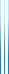# PublicationsManuscripts

## Matrix multiplication

Yuval Filmus

An exposition on algorithms for matrix multiplication, in two parts:

• Part 1:
• The arithmetic model.
• Bilinear normal form for matrix multiplication.
• Tensor notation and tensor rank.
• Border rank.
• Schönhage’s $\tau$ theorem (the asymptotic sum inequality).
• Coppersmith’s $\tilde{O}(n^2)$ algorithm for multiplying rectangular matrices.
• Part 2:
• The laser method.